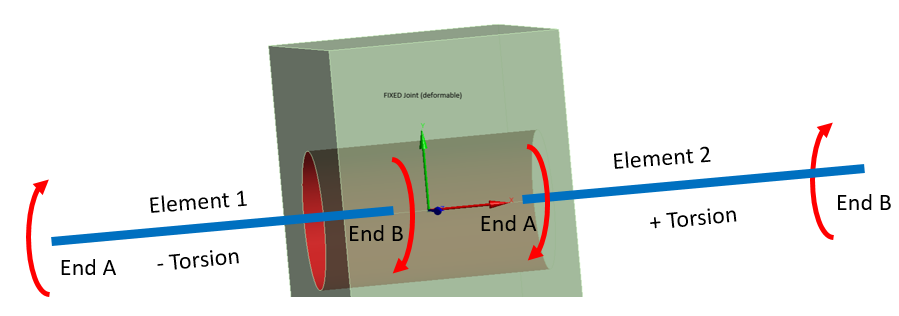## General Mechanical

Topics relate to Mechanical Enterprise, Motion, Additive Print and more

•Rameez_ul_Haq
Subscriber

Observe the simple model below.

•peteroznewman
Subscriber
nI understand Example 1. A cylindrical rod is supporting a lever arm, and the force on the end of the lever creates bending, shear and torsion in the rod by a single Fixed Joint in the center of the hole. Fine.nI don't understand Example 2. It seems you still have a cylindrical rod and a lever arm, but now you are playing games splitting faces 25/75% in the hole. Are you using two joints? This makes no practical sense. What is the physical example of this?n
•Rameez_ul_Haq
Subscriber
,well to be honest, it is kind of like playing games in order to understand how would a joint work in different conditions. Near me, this is the best I can do to thoroughly understand how would a joint behave, how would the transfer of forces and moments to the line body's vertices occur. If I am able to grasp this, using the joint at the correct locations would become much more clearer and the modelling would be more accurate.nYes, there are two separate joints. Imagine, I dont want to model a bolt as a solid body. I want to model it as like a line body, for obvious reasons (i.e. to decrease the number of total number of elements within the whole model). Now, if I use a single joint between the face and a middle vertex of the line body, then all the force transfer from the face will only be to that single vertex of the line body. But in case of the bolt modelled as a solid body [first by establishing appropriate connections bewteen the hole's and bolt's faces], this is not true. The transfer of forces from the hole's face can change along the line of the bolt (depending upon how is the hole behaving along its length). To model this situation, what I can do is that I can break the hole's faces into many many faces, and then establish a fixed joint between each of the face with each of the vertex of the line body. This would mean that should be a difference between the force transfer between, for example, two adjacent fixed joints established (although the total amount of force transfer will remain the same). To ensure this believe, I tried to conduct an initial analysis if this would be true. But unfortunately, the force transfer within each of the joint still is the same. This confuses me. nI hope I was able to clarify my query. n
•peteroznewman
Subscriber
nA lever with a hole in it is assembled onto a rod and the lever is fixed to the rod so that forces at the end of the lever can be transferred into the rod, and presumably, onto other parts that do useful things. I might use a fixed joint to connect the lever to the rod because it is fast and it allows a model to be built quickly to assess the overall design. What will be learned from this first simplified model is where areas of concern are in the design, so more detail can be put into the model to revise the answers.nIf it turned out that the area of concern was where the rod is fastened to the lever, that would justify replacing the beam element with a solid body and putting in the detail of how the rod and lever are fastened together. They may be welded, maybe there is a set-screw tightened onto a flat, or a dowel pin in a cross-wise slot. Maybe several of these methods are evaluated.nI would not slice up the face of the hole and add more fixed joints. That is not helpful.n
•Rameez_ul_Haq
Subscriber
,I understand sir. But sir think, and as seen in the both of the above examples where the force reaction in both of the fixed supports is equal, and if this is a substructure of a big model, and then I have to put these reaction forces onto each of the other parts where I have modelled them as fixed supports; I would be putting equal forces at both of those locations. Even though the area of concern is not where the rod is fastened to the lever, but the force transfer to each of fixed supports is important since those regions might be extremely sensitive to the amount of force transfer to them. nCan you tell me why is the torsional force equal but opposite at both of the fixed supports in example 1? I was hoping for them to be equal, and also in the same direction.n
•peteroznewman
Subscriber
nWhat do you mean the same direction? Torsional moment has a sign convention. A beam element has an End A and an End B. The beam axis is defined to go from A to B. Use the Right-Hand Rule to define a positive torque at end B. The diagram below shows two elements with a positive and negative torsion moment. If you want the torsional moment to have the same sign, then swap the nodes on element 1, the axis will go in the other direction and the torsional moment will change sign.nn
•Rameez_ul_Haq
Subscriber
,how can I the swap the nodes of a beam element, or in other words reverse the element orientation of an element in ANSYS?nAnd what does 'Linearized Joint Behavior' mean?n
•peteroznewman
Subscriber
nIf you have three nodes, 1, 2 and 3 and you were defining the elements using APDL, then instead of E,1,2 you would type E,2,1 while the other element would be E,2,3.nI don't know how to do that in Mechanical. I don't know if you draw two lines from the center to the ends, does that swap End A and B.nLinearized Joint Behavior means small displacement, small rotation assumptions apply.n